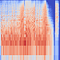Data CollectionMel Spectrogram image of the song “A Day In The Life” off Sgt. Pepper’s Lonely Hearts Club

Interpreting the Results

from model_staging import fetch_images_dataframe
import pandas as pd
import numpy as np
import keras
train_path = "C://Users//Alec//MyPython//Beatles/train_melspec"
test_path = "C://Users//Alec//MyPython//Beatles/test_melspec"
# load the Beatles data to predict on
test_gen = fetch_images_dataframe(test_df, x_col="song",
y_col="artist",
directory=test_path,
batch_size=16,
target_size=(256, 256),
class_mode="categorical",
shuffle=False, seed=1,
save_format="png")
# get the prediction based on largest probability
preds = np.argmax(probabilities, axis=1)
# load in the training data to get the artist indicies
train_gen, valid_gen = fetch_images_dataframe(train_df,
x_col="song",
y_col="artist",
directory=train_path,
batch_size=16,
target_size=(256, 256),
class_mode="categorical",
shuffle=True, seed=1,
validation_split=0.2,
save_format="png")
class_map = train_gen.class_indices
# create a dataframe of songs and predictions
pred_df = pd.DataFrame(data={"songs": test_gen.filenames,
"predictions": preds})
# now convert the prediction column to the artist name
mapping = {v:k for k,v in class_map.items()}
pred_df["predictions"] = pred_df["predictions"].map(mapping)
# merge the pred_df with the test_df in order to bring the album
# name in for each song
pred_df = pred_df.merge(test_df[["album", "song"]], left_on="songs",right_on="song")
pred_df.drop("song", axis=1, inplace=True)
# join the prediction probabilities with the prediction dataframe
pred_df = pred_df.join(pd.DataFrame(probabilities))
pred_df.rename(mapping, axis=1, inplace=True)
pred_df[['Lennon', 'harrison', 'mccartney', 'starr']] = pred_df[['Lennon', 'harrison', 'mccartney', 'starr']].round(4)

Conclusion and Lessons Learned

Applied Data Science Master's Student. Data Science and finance enthusiast,

More from Alec Francis

Applied Data Science Master's Student. Data Science and finance enthusiast,